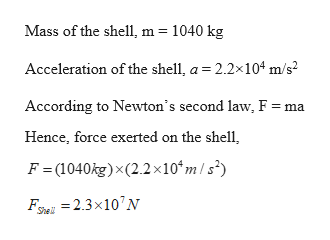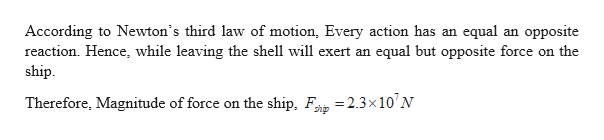A 1040 kg artillery shell is fired from a battleship. While it is in the barrel of the gun, it experiences an acceleration of 2.2 × 104 m/s2.m=1040 kga = 2.2 × 104 m/s2Part (a)  What net force is exerted on the artillery shell before it leaves the barrel of the gun (in Newtons)? Part (b)  What is the magnitude of the force exerted on the ship by the artillery shell in Newtons?

Question

A 1040 kg artillery shell is fired from a battleship. While it is in the barrel of the gun, it experiences an acceleration of 2.2 × 104 m/s2.

m=1040 kg

a = 2.2 × 104 m/s2

Part (a)  What net force is exerted on the artillery shell before it leaves the barrel of the gun (in Newtons)?

Part (b)  What is the magnitude of the force exerted on the ship by the artillery shell in Newtons?

Step 1

Given:

m = 1040 kg

a = 2.2×104 m/s2

Step 2

(a) Calculating the net force is exerted on the artillery shell before it leaves the barrel of the gun:help_outlineImage TranscriptioncloseMass of the shell, m 1040 kg Acceleration of the shell, a 2.2x104 m/s2 According to Newton's second law, F = ma Hence, force exerted on the shell F = (1040kg)x(2.2x10*m /s') F=2.3x10N She fullscreen
Step 3

(b) Calculating the magnitude of the force exerted...help_outlineImage TranscriptioncloseAccording to Newton's third law of motion, Every action has an equal an opposite reaction. Hence, while leaving the shell will exert an equal but opposite force on the ship Therefore, Magnitude of force on the ship, F2.3x 10 N shp fullscreen

Want to see the full answer?

See Solution

Want to see this answer and more?

Our solutions are written by experts, many with advanced degrees, and available 24/7

See Solution
Tagged in

Newtons Laws of Motion